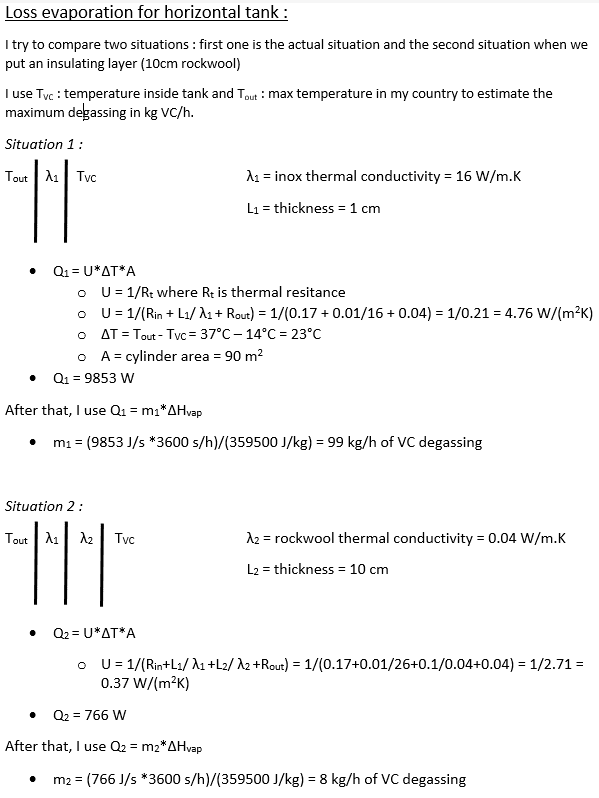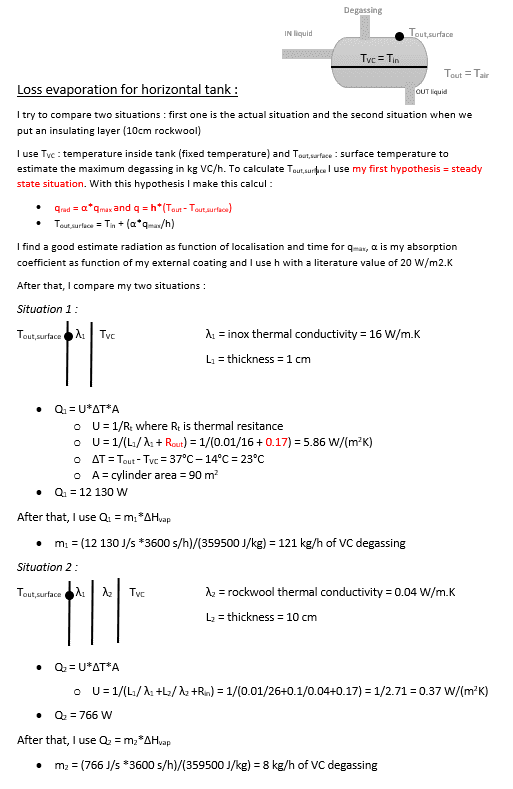# Solar radiation on a horizontal tank

eclaisse
Hello everyone, I'm looking to estimate the variation of degassing on a horizontal tank of VC (vinyl chloride) due to solar radiation.
The feed flow is continuous but the outlet is batch flow. So there is a lot of variation of liquid level in the tank (constant pressure).
To avoid a lot of degassing during big heats, I want to estimate if it is a good idea to put an insulator around the tank.

How can I include a insulating element in my calculation ?
For now, I just have the basic equation of energy balance (convection and solar radiation).

Thanks.

Mentor
Let's see what you've done so far.

•BvU
Welcome to PF.

What is your approximate latitude on Earth ?
Is the tank axis N-S or E-W ?

How much variation is there in ambient air temperature ?
What exactly do you mean by “big heats” ?

What is the maximum capacity of the tank ?

•eclaisse
eclaisse
Thanks Baluncore.

Here is some information :
Approximate latitude : 51° (Belgium) and the tank axis is N-S with a diameter of 3m and a length of 10 meters.
I want to see the utility of insulating. During summer we have temperature around 30 degrees celsius.

I try to use the basic equation : Q = U*deltaT*A where U = 1/R = 1/hi + lambda/e + 1/he
Then I compare with the addition of 10 cm rockwool as isolation.
So finally I have Q= [T(outside)-T(inside)]*U*A and I can compare and see the difference between both situation.

I have some questions :
1) I use basic data for hi and he (0.17 et 0.04 m^2.K/W) => Is it ok ?
2) Can I include the solar radiation with this method ?
3) After that, Can I use Q=m*Hvap to estimate my degassing ?

PS : I will upload my calculation in few hours (it will be clearer...)

Last edited:
eclaisse
After some research, I do these calculations...
It looks okai. But I still need to include the effect of solar radiation...Homework Helper
Is the VC at ##\ \ +##14 ##^\circ##C ? or at ##\ \ -##14 ?

eclaisse
It is +14°C. It is a calculated value (saturation temperature) from data pressure (2.8bar)

•BvU
eclaisse
Hello, I update my calcul if someone is interested.
I juste have two questions : My way to estimate my outside surface temperature seems okay ?
And I have a big problem to estimate an average convection coefficient for both situation : (h_out in my first calculation (1/h_out = 0.05 m2.K/W) and h_in in my second (1/h_in = 0.17 m2.K/W). These coefficients change a lot the final result and I am not sure about my values (I just use theoretical value find in literature...).

Thanks a lot.#### Attachments

Mentor
The key to this is to look at a worst case scenario, using upper bounds to the inside and outside heat transfer coefficients. That way the calculation should be conservative, and give an upper bound to the evaporation rate. I think your value for the inside heat transfer coefficient looks low. I would use a much higher value, on the order 500. Your outside heat transfer coefficient looks reasonable. Considering that, in the actual situation, the insulation is going to dominate, I guess these values wouldn't matter much.